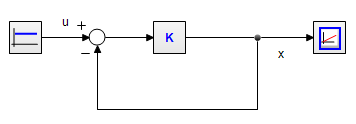﻿ 20-sim webhelp > Editor > Compiling > Algebraic Loops

# Algebraic Loops

An algebraic loop in a model is a loop consisting of elements without "memory like" functions. To calculate the variables in this loop, the variable values themselves are needed. Consider the following example of an algebraic loop in an amplifier with negative feedback:Standard derivation of a simulation model would yield:

x = K*(u-x)

The variable x depends on its own value and must be solved by iteration. In 20-sim every simulation algorithm is accompanied by an iteration routine. Fortunately 20-sim is able to solve many algebraic loops at equation level. For this model this leads to the analytic solution the system:

x = K*u/(1+K)

## Simulating algebraic loops

Although 20-sim contains a sophisticated algorithms to find analytic solutions, the occurrence of unbreakable loops can not always be prevented. The occurrence of algebraic loops may lead to an increase of simulation time, or even stop simulation when iteration fails.

The best solution for these problems is to have a critical look at the model and change the order of calculations in a model. Possible solutions are:

 • Algebraic Loops occur when the order of calculations is arbitrary. When an algebraic loop occurs in an equation model or in a set of equation models, you may change the order of calculation by rewriting the equations. The calculation order in bond graph models can be changed by introducing hand-defined causality.
 • Introduce ‘parasitic’ energy storage elements (e.g. a small mass, a small capacitor etc.) to break an algebraic loop. These elements introduce however, large poles in the state equations, which might increase the simulation time considerably.
 • Delete elements in the algebraic loop which are not relevant for the model’s simulation output (e.g. small dampers, very stiff springs etc.). Care should however be taken, since correct deletions are not always possible and require considerable modeling skill and intuition.
 • Combine dual elements. Sometimes elements of the same type can be combined by adding the parameter values (e.g. combining a mass m1 and a mass m2 to a mass m1 + m2). This will in most cases decrease the amount of algebraic loops.